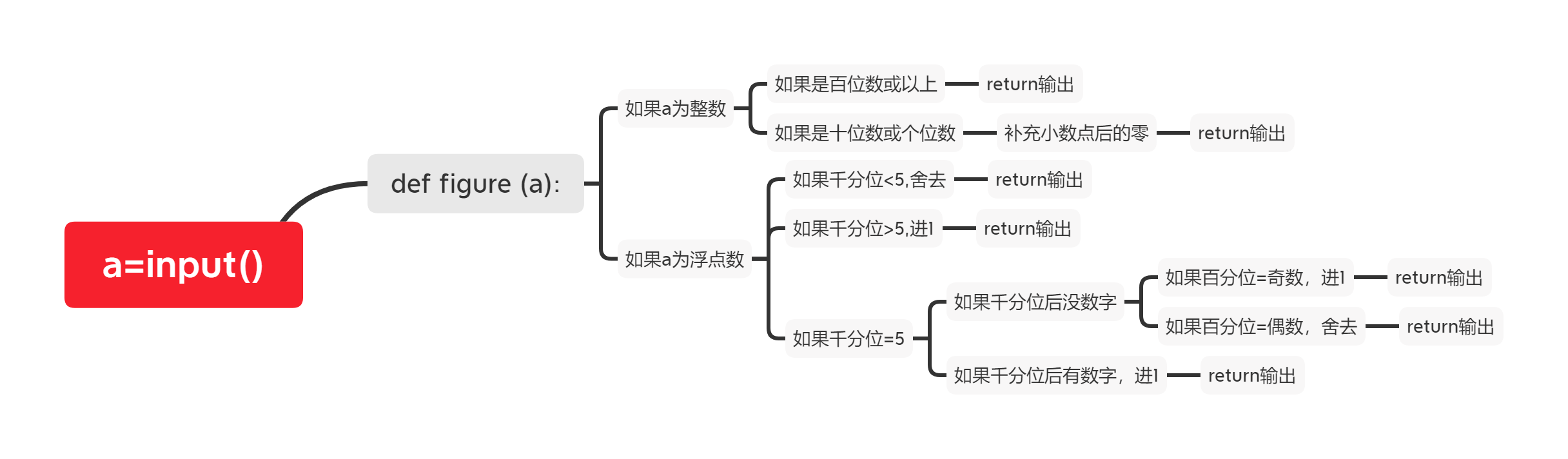2021-04-28 12:04

# 用python实现保留3位有效数字（四舍六入五成双规则）12 12.0

4 4.00

0.2 0.200

0.32 0.320

1.3 1.30

1.235 1.24

1.245 1.24

1.2451 1.25

• 写回答
• 好问题 提建议
• 追加酬金
• 关注问题
• 邀请回答

#### 4条回答默认 最新

•kaili_ya 2021-04-28 15:11
最佳回答
``````a = input()
if '.' in a:
a = float(a)
if a*1000%10!=5:
a = '%.2f'%(a)
else:
if len(str(a).split("."))>3:
a = '%.2f'%(a)
else:
if int(a*100%10%2)==1:
a = float('%.2f'%float(int(a*100)/100))+0.01
else:
a = '%.2f'%float(int(a*100)/100)
print(a)
else:
a = int(a)
if a>99:
print(a)
else:
print(float(a))``````
评论
解决 无用
打赏 举报